# Selina Concise Solutions for Chapter 16 Understanding Shapes Class 8 ICSE Mathematics

##### Exercise 16A

1. State which of the following are polygons:If the given figure is a polygon, name it as convex or concave.
Solution
Only Fig. (ii), (iii) and (v) are polygons.
Fig. (ii) and (iii) are concave polygons while
Fig. (v) is convex.

2. Calculate the sum of angles of a polygon with :
(i) 10 sides
(ii) 12 sides
(iii) 20 sides
(iv) 25 sides
Solution
(i) No. of sides n = 10
sum of angles of polygon = (n – 2)×180°
= (10 – 2)× 180° = 1440°

(ii) no. of sides n = 12
sum of angles = (n – 2)×180°
= (12 – 2)×180° = 10×180° = 1800°

(iii) n = 20
Sum of angles of Polygon = (n – 2)× 180°
= (20 – 2)× 180° = 3240°

(iv) n = 25
Sum of angles of polygon = (n – 2)× 180°
= (25 – 2)× 180° = 4140°

3. Find the number of sides in a polygon if the sum of its interior angles is :
(i) 900°
(ii) 1620°
(iii) 16 right-angles
(iv) 32 right-angles.
Solution
(i) Let no. of sides = n
Sum of angles of polygon = 900˚
(n – 2) × 180˚ = 900˚
⇒ n – 2 = 900/180
⇒ n – 2 = 5
⇒ n = 5 + 2
⇒ n = 7

(ii) Let no. of sides = n
Sum of angles of polygon = 1620˚
(n – 2) × 180˚ = 1620˚
⇒ n – 2 = 1620/180
⇒ n – 2 = 9
⇒ n = 9 + 2
⇒ n = 11

(iii) Let no. of sides = n
Sum of angles of polygon = 16 right = 16 × 90 = 1440˚
(n – 2) × 180˚ =  1440˚
⇒ n – 2 = 1440/180˚
⇒ n – 2 = 8
⇒ n = 8 + 2
⇒ n = 10

(iv) Let no. of sides = n
Sum of angles of polygon = 32 right angles = 32 × 90 = 2880˚
(n × 2) × 180˚ = 2880
n – 2 = 2880/180
n – 2 = 16
n = 16 + 2
n =18

4. Is it possible to have a polygon; whose sum of interior angles is :
(i) 870°
(ii) 2340°
(iii) 7 right-angles
(iv) 4500°
Solution
(i) Let no. of sides = n
Sum of angles = 870°
(n – 2) × 180° = 870°
⇒ n – 2 = 870/180
⇒ n – 2 = 29/6
⇒ n = 29/6 + 2
⇒ n = 41/6
Which is not a whole number.
Hence it is not possible to have a polygon, the sum of whose interior angles is 870°

(ii) Let no. of sides = n
Sum of angles = 2340°
(n – 2) × 180° = 2340°
⇒ n – 2 = 2340/180
⇒ n – 2 = 13
⇒ n = 13 + 2 = 15
Which is a whole number.
Hence it is possible to have a polygon, the sum of whose interior angles is 2340°.

(iii) Let no. of sides = n
Sum of angles = 7 right angles = 7 ×90 = 630°
(n – 2) × 180° = 630°
⇒ n – 2 = 630/180
⇒ n – 2 = 7/2
⇒ n = 7/2 + 2
⇒ n = 11/2
Which is not a whole number. Hence it is not possible to have a polygon, the sum of whose interior angles is 7 right-angles.

(iv) Let no. of sides = n
(n – 2)×180° = 4500°
⇒ n – 2 = 4500/180
⇒ n – 2 = 25
⇒ n = 25 + 2
⇒ n = 27
Which is a whole number.
Hence it is possible to have a polygon, the sum of whose interior angles is 4500°.

5. (i) If all the angles of a hexagon are equal; find the measure of each angle.
(ii) If all the angles of a 14-sided figure are equal; find the measure of each angle.
Solution
(i) No. of sides of hexagon, n = 6
Let each angle be = x°
Sum of angles = 6x°
(n – 2)× 180° = Sum of angles
⇒ (6 – 2)× 180° = 6x°
⇒ 4× 180 = 6x
⇒ x = (4 × 180)/6
⇒ x = 120˚
∴ Each angle of hexagon = 120˚

(ii) No. of sides of polygon,  n = 14
Let each angle = x˚
∴ Sum of angles = 14x˚
∴ (n – 2) × 180˚ = Sum of angles of polygon
∴ (14 – 2) × 180˚ = 14x
12 × 180˚ = 14x
⇒ x = (12 × 180)/14
⇒ x = 1080/7
⇒ x = (154 2/7)˚

6. Find the sum of exterior angles obtained on producing, in order, the sides of a polygon with :
(i) 7 sides
(ii) 10 sides
(iii) 250 sides.
Solution
(i) No. of sides n = 7
Sum of interior & exterior angles at one vertex = 180°
Sum of interior & exterior angles = 7 × 180˚
= 1260˚
Sum of interior angles = (n – 2) × 180˚
= (7 – 2) × 180˚
= 900˚
∴ Sum of exterior angles = 1260˚ - 900˚
= 360˚

(ii) No. of sides n = 10
Sum of interior and exterior angles = 10 × 180˚
= 1800˚
But sum of interior angles = (n – 2) × 180˚
= (10 – 2) × 180˚
= 1440˚
∴ Sum of exterior angles = 1800 – 1440
= 360˚

(iii) No. of  sides n = 250
Sum of all interior and exterior angles = 250 × 180˚
= 45000˚
But sum of interior angles = (n – 2) × 180˚
= (250 – 2) × 180˚
= 248 × 180˚
= 44640˚
∴  Sum of exterior angles = 45000 – 44640
= 360˚

7. The sides of a hexagon are produced in order. If the measures of exterior angles so obtained are (6x – 1)°, (10x + 2)°, (8x + 2)° (9x – 3)°, (5x + 4)° and (12x + 6)° ; find each exterior angle.
Solution
Sum of exterior angles of hexagon formed by producing sides of order = 360°
∴ (6x – 1)˚ - (10x + 2)˚ + (8x + 2)˚ + (9x – 3)˚ + (5x + 4)˚ + (12x + 6)˚  = 360˚
50x + 10˚ = 360˚
⇒ 50x = 360˚ - 10˚
⇒ 50x = 350˚
⇒ x = 350/70
⇒ x = 7
∴ Angles are (6x – 1)˚ : (10x + 2)˚ : (8x + 2)˚ : (9x – 3)˚ : (5x + 4)˚ and (12x + 6)˚
i.e.  (6 × 7 – 1)˚ : (10 × 7 + 2)˚ : (8 × 7 + 2)˚ : (9 × 7 – 3)˚ : (5 × 7 + 4)˚ : (12 × 7 + 6)˚
i.e. 41° ; 72°, 58° ; 60° ; 39° and 90°

8. The interior angles of a pentagon are in the ratio 4: 5: 6: 7: 5. Find each angle of the pentagon.
Solution
Let the interior angles of the pentagon be 4x, 5x, 6x, 7x, 5x.
Their sum = 4x + 5x + 6x + 7x + 5x = 21x
Sum of interior angles of a polygon = (n – 2) × 180˚
= (5 – 2) × 180˚
= 540˚
∴ 27x = 540
⇒ x = 540/27
⇒ x = 20˚
∴ Angles are 4 × 20˚ = 80˚
5 × 20˚ = 100˚
6 × 20˚ = 120˚
7 × 20˚ = 140˚
5 × 20˚ = 100˚

9. Two angles of a hexagon are 120° and 160°. If the remaining four angles are equal, find each equal angle.
Solution
Two angles of a hexagon are 120°, 160°
Let remaining four angles be x, x, x and x.
Their sum = 4x + 280°
But sum of all the interior angles of a hexagon
= (6 – 2) × 180˚
= 4 × 180˚
= 720˚
∴ 4x  + 280˚ = 720˚
⇒ 4x = 720˚ - 280˚
⇒ 4x = 440˚
⇒ x = 110˚
∴ Equal angles are 110˚ (each)

10. The figure, given below, shows a pentagon ABCDE with sides AB and ED parallel to each other, and ∠B: ∠C : ∠D = 5: 6: 7.(i) Using formula, find the sum of interior angles of the pentagon.
(ii) Write the value of ∠A + ∠E
(iii) Find angles B, C and D.
Solution
(i) Sum of interior angles of the pentagon
= (5 – 2) × 180˚
= 3 × 180˚ = 540˚  [∵ sum for a polygon of x sides = (x – 2) × 180˚]

(ii) Since AB ∥ ED
∴ ∠A + ∠E = 180˚

(iii) Let  ∠B = 5x   ∠C = 6x    ∠D = 7x
∴ 5x + 6x + 7x + 180˚ = 540˚ (∠A + ∠E = 180˚) Proved in (ii)
⇒ 18x = 540˚ - 180˚
⇒ 18x = 360˚
⇒ x = 20˚
∴ ∠B = 5 × 20˚ = 100˚,
∠C = 6 × 20˚ = 120˚
∠D = 7 × 20 = 140˚

11. Two angles of a polygon are right angles and the remaining are 120° each. Find the number of sides in it.
Solution
Let number of sides = n
Sum of interior angles = (n – 2) × 180˚
= 180n - 360˚
Sum of 2 right angles = 2 × 90˚
= 180˚
∴ Sum of other angles = 180˚n - 360˚ - 180˚
= 180˚n - 540˚
No. of vertices at which these angles are formed = n – 2
∴ Each interior angle = (180n – 540)/(n – 2)
∴ (180n – 540)/(n – 2) = 120˚
⇒ 180n – 540 = 120n – 240
⇒ 180n – 120n = -240 + 540
⇒ 60n = 300
⇒ n = 300/60
⇒ n = 5

12. In a hexagon ABCDEF, side AB is parallel to side FE and ∠B: ∠C: ∠D: ∠E = 6 : 4: 2: 3. Find ∠B and ∠D.
Solution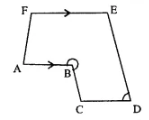Given: Hexagon ABCDEF  in which AB∥ EF and ∠B: ∠C: ∠D: ∠E = 6: 4: 2 : 3.
To find: ∠B and ∠D
Proof: No. of sides n = 6
∴ Sum of interior angles = (n – 2) × 180˚
= (6 – 2) × 180˚
= 720˚
∵ AB ∥ EF (Given)
∴ ∠A + ∠F = 180˚
But ∠A + ∠B + ∠C + ∠D + ∠E + ∠F = 720˚  (Proved)
∠B + ∠C + ∠D + ∠E + ∠180˚ = 720˚
∴ ∠B + ∠C + ∠D + ∠E = 720˚ - 180˚ = 540˚
Ratio = 6 : 4 : 2 : 3
Sum of parts = 6 + 4 + 2 + 3  = 15
∴ ∠B = 6/15 × 540 = 216˚
∠D = 2/15 × 540 = 72˚
Hence, ∠B = 216˚; ∠D = 72˚

13. The angles of a hexagon are x + 10°, 2x + 20°, 2x – 20°, 3x – 50°, x + 40° and x + 20°. Find x.
Solution
Angles of a hexagon are x + 10˚, 2x + 20˚, 2x - 20˚, 3x - 50˚, x + 40˚ and  x + 40˚ and x + 20˚
∴ But sum of angles of a hexagon = (x – 2) × 180˚
= (6 – 2) × 180˚
= 4 × 180˚
= 720˚
But sum = x + 10 + 2x + 20˚ + 2x - 20˚ + 3x - 50˚ + x + 40 + x + 20
= 10x + 90 – 70
= 10x + 20
∴ 10x + 20 = 720˚
⇒ 10x = 72 - 20 = 700
⇒ x = 700˚/10 = 70˚
∴ x = 70˚

14. In a pentagon, two angles are 40° and 60°, and the rest are in the ratio 1 : 3: 7. Find the biggest angle of the pentagon.
Solution
In a pentagon, two angles are 40° and 60° Sum of remaining 3 angles = 3× 180°
= 540° – 40° – 60° = 540° – 100° = 440°
Ratio in these 3 angles =1 : 3 : 7
Sum of ratios =1 + 3 + 7 = 11
Biggest angle = (440 × 7)/11 = 280°

### Exercise 16 B

1.Fill in the blanks :
In case of regular polygon, with :SolutionExplanation
(i) Each exterior angle = 360˚/8 = 45˚
Each interior angle = 180˚ - 45˚ = 135˚

(ii) Each exterior angle = 360˚/12 = 30˚
Each interior angle = 180˚ - 30˚ = 150˚

(iii) Since each exterior = 72˚
∴ Number of sides = 360˚/72˚ = 5
Also interior angle = 180˚ - 72˚ = 108˚

(iv) Since each exterior angle = 45˚
∴ Number of sides = 360˚/45˚ = 8
Interior angle = 180˚ - 45˚ = 135˚

(v) Since interior angle = 150˚
∴ Exterior angle = 180˚ - 150˚ = 30˚
∴ Number of sides = 360˚/30˚ = 12

(vi) Since interior  angle = 140˚
∴ Exterior angle = 180˚ - 140˚ = 40˚
∴ Number of sides = 360˚/40˚ = 9

2. Find the number of sides in a regular polygon, if its each interior angle is :
(i) 160°
(ii) 135°
(iii) 1 1/5 of a right-angle
Solution

(i) Let no. of sides of regular polygon be n.
Each interior angle = 160˚
∴ (n – 2)/n × 180˚ = 360˚
⇒ 180˚n - 360˚ = 160n
⇒ 20n = 360˚
⇒ n = 18

(ii) No. of  sides = n
Each interior angle = 135˚
(n – 2)/n × 180˚ = 135˚
⇒ 180n - 360˚ = 135n
⇒ 180n – 135n = 360˚
⇒ 45n = 360˚
⇒ n = 8

(iii) No. of sides = n
Each interior angle = 1 1/5 right angles
= 6/5 × 90˚
= 108˚
∴ (n – 2)/n × 180˚ = 108˚
⇒ 180n – 360 ˚ = 108n
⇒ 180n -108n = 360˚
⇒ 72n = 360˚
⇒ n = 5

3. Find the number of sides in a regular polygon, if its each exterior angle is :
(i) 1/3 of a right angle
(ii) two-fifth of a right-angle.
Solution
(i) Each exterior angle = 1/3  of a right angle
= 1/3 ×90
= 30°
Let number of sides = n
∴ 360˚/n = 30˚
∴ n = 360˚/30˚
⇒ n = 12

(ii) Each exterior angle = 2/5 of a right-angle
= 2/5 × 90˚
= 36˚
Let number of sides = n
∴ 360˚/n = 36˚
⇒ n = 360˚/36˚
⇒ n = 10

4. Is it possible to have a regular polygon whose each interior angle is :
(i) 170°
(ii) 138°
Solution
(i) No. of sides = n
each interior angle = 170°
∴ (n – 2)/n × 180˚  = 170˚
⇒ 180n - 360˚ = 170n
⇒ 180n – 170n = 360˚
⇒ 10n = 360˚
⇒ n = 360˚/10˚
⇒ n = 36
which is a whole number.
Hence it is possible to have a regular polygon whose interior angle is 170˚.

(ii) Let no. of sides = n
Each interior angle = 138˚
∴ (n – 2)/n × 180˚ = 138˚
⇒ 180n - 360˚ = 138n
⇒ 180n – 138n = 360˚
⇒ 42n = 360˚
⇒ n = 360˚/42
⇒ n = 60˚/7
Which is not a whole number.
Hence it is not possible to have a regular polygon having interior angle of 138°.

5. Is it possible to have a regular polygon whose each exterior angle is :
(i) 80°
(ii) 40% of a right angle.
Solution
(i) Let no. of sides = n each exterior angle = 80°
360˚/n = 80˚
⇒ n = 360˚/80˚
⇒ n = 9/2
Which is not a whole number.
Hence it is not possible to have a regular polygon whose each exterior angle is of 80°.

(ii) Let number of sides = n
Each exterior angle = 40% of a right angle
= 40/100 × 90
= 36˚
n = 360˚/36˚
⇒ n = 10
Which is a whole number.
Hence it is possible to have a regular polygon whose each exterior angle is 40% of a right angle.

6. Find the number of sides in a regular polygon, if its interior angle is equal to its exterior angle.
Solution
Let each exterior angle or interior angle be = x°∴ x + x = 180˚
⇒ 2x = 180˚
⇒ x = 90˚
Now, let no. of sides = n
∵ Each exterior angle = 360˚/n
∴ 90˚ = 360˚/n
⇒ n = 360˚/90˚
⇒ n = 4

7. The exterior angle of a regular polygon is one-third of its interior angle. Find the number of sides in the polygon.
Solution
Let interior angle = x°
Exterior angle = 1/3 x°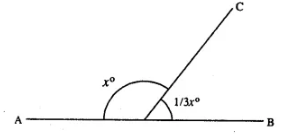∴ x + 1/3x = 180˚
⇒ 3x + x = 540
⇒ 4x = 540
⇒ x = 540/4
⇒ x = 135˚
∴ Exterior angle = 1/3 × 135˚ = 45˚
Let no. of sides = n
∵ Each exterior angle = 360˚/n
∴ 45˚ = 360˚/n
∴ n = 360˚/45˚
⇒ n = 8

8. The measure of each interior angle of a regular polygon is five times the measure of its exterior angle. Find :
(i) measure of each interior angle ;
(ii) measure of each exterior angle and
(iii) number of sides in the polygon.
Solution
Let exterior angle = x°
Interior angle = 5x°
x + 5x = 180°
⇒ 6x = 180°
⇒ x = 30°
Each exterior angle = 30°
Each interior angle = 5 x 30° = 150°
Let no. of sides = n
∵ each exterior angle = 360˚/n
30˚ = 360˚/n
⇒ n = 360˚/30˚
⇒ n = 12
Hence, (i) 150˚ (ii) 30˚ (iii) 12

9. The ratio between the interior angle and the exterior angle of a regular polygon is 2 : 1. Find :
(i) each exterior angle of the polygon ;
(ii) number of sides in the polygon
Solution
Interior angle : exterior angle = 2 : 1
Let interior angle = 2x° & exterior angle = x°∴ 2x˚ + x˚ = 180˚
⇒ 3x = 180˚

(i) x = 60˚
∴ Each exterior angle = 60˚
Let angle of sides = n
∴ 360˚/n = 60˚
⇒ n = 360˚/60˚
⇒ n = 360˚/60˚

(ii) n = 6
∴ (i) 60˚  (ii) 6

10. The ratio between the exterior angle and the interior angle of a regular polygon is 1: 4. Find the number of sides in the polygon.
Solution
Let exterior angle be x° & interior angle be 4x°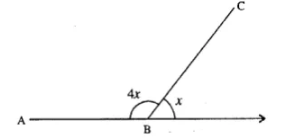∴ 4x + x = 180˚
⇒ 5x = 180˚
⇒ x = 36˚
∴ Each exterior angle = 36˚
Let no. sides = n
∴ 360˚/n = 36˚
⇒ n = 360˚/36
⇒ n = 10

11. The sum of interior angles of a regular polygon is twice the sum of its exterior angles. Find the number of sides of the polygon.
Solution
Let number of sides = n
Sum of exterior angles = 360°
Sum of interior angles = 360° × 2 = 720°
Sum of interior angles = (n – 2) × 180°
720° = (n – 2)× 180°
⇒ n – 2 = 720/180
⇒ n – 2 = 4
⇒ n = 4 + 2
⇒ n = 6

12. AB, BC and CD are three consecutive sides of a regular polygon. If angle BAC = 20° ; find :
(i) its each interior angle,
(ii) its each exterior angle
(iii) the number of sides in the polygon.
Solution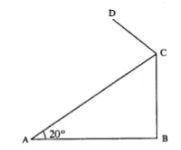∵ Polygon is regular  (Given)
∴ AB = BC
⇒ ∠BAC = ∠BAC  [∠s opp.  to equal sides]
But   ∠BAC = 20˚
∴ ∠BCA = 20˚
i.e., In ΔABC,
∠B + ∠BAC + ∠BCA = 180˚
⇒ ∠B + 20˚ + 20˚ = 180˚
⇒ ∠B = 180˚ - 40˚
⇒ ∠B = 140˚
(i) each interior angle = 140˚
(ii) each exterior angle = 180˚ - 140˚ = 40˚
(iii) Let no. of sides = n
∴ 360˚/n = 40˚
⇒ n = 360˚/40˚ = 9
⇒ n = 9
∴ (i) 140˚ (ii) 9

13. Two alternate sides of a regular polygon, when produced, meet at the right angle. Calculate the number of sides in the polygon.
Solution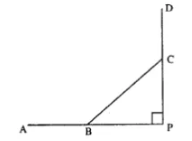Let number of sides of regular polygon = n
AB & DC when produced meet at P such that
∠P = 90˚
∵ Interior angles are equal.
∴ ∠ABC = ∠BCD
∴ 180˚ - ∠ABC = 180˚ - ∠BCD
∴ ∠PBC = ∠BCP
But   ∠P = 90˚  (Given)
∴ ∠PBC + ∠BCP = 180˚ - 90˚ = 90˚
∴ ∠PBC = ∠BCP
= ½ × 90˚
= 45˚
∴ Each exterior angle = 45˚
∴ 45˚ = 360˚/n
⇒ n = 360˚/45˚
⇒ n = 8

14. In a regular pentagon ABCDE, draw a diagonal BE and then find the measure of:
(i) ∠BAE
(ii) ∠ABE
(iii) ∠BED
Solution
(i) Since number of sides in the pentagon = 5
Each exterior angle = 360/5 = 72°
∠BAE = 180° – 72°= 108°(ii) In ΔABE, AB = AE
∴ ∠ABE  = ∠AEB
But ∠BAE + ∠ABE + ∠AEB = 180˚
∴ 108˚ + 2∠ABE
= 180˚ - 108˚
= 72˚
⇒ ∠ABE = 36˚

(iii) Since ∠AED = 108˚
[∵ each interior angle = 108˚]
⇒ ∠AEB = 36˚
⇒ ∠BED = 108˚ - 36˚
= 72˚

15. The difference between the exterior angles of two regular polygons, having the sides equal to (n – 1) and (n + 1) is 9°. Find the value of n.
Solution
We know that sum of exterior angles of a polynomial is 360°

(i) If sides of a regular polygon = n – 1
Then each angle = 360˚/(n – 1)
And if sides are n + 1, then each angle = 360˚/(n +1)
According to the condition,
360˚/(n – 1) - 360˚/(n + 1) = 9
⇒ 360˚[1/(x - 1) - 1/(x + 1)] = 9
⇒ 360˚ [(n + 1 – n +  1)/(n – 1)(n + 1)] = 9
⇒ (2 × 360)/(n2 – 1) = 9
⇒ n2 – 1 = (2×360)/9 = 80
⇒ n2 – 1  = 80
⇒ n2 = 1 – 80 = 0
⇒ n2 – 81 = 0
⇒ (n)2 – (9)2 = 0
⇒ (n + 9)(n – 9) = 0
Either n + 9 = 0, then n = -9 which is not possible being negative,
Or, n – 9 = 0, then  n = 9
∴ n = 9
∴ No. of sides of a regular polygon = 9

16. If the difference between the exterior angle of a n sided regular polygon and an (n + 1) sided regular polygon is 12°, find the value of n.
Solution
We know that sum of exterior angles of a polygon = 360°
Each exterior angle of a regular polygon of 360°
sides = 360˚/n
And exterior angle of the regular polygon of  (n + 1) sides = 360˚/(n + 1)
∴ 360˚/n - 360˚/(n + 1) = 12
⇒ 360 [1/n – 1/(n + 1)] = 12
⇒ 360[(n + 1 – n)/n(n + 1)] = 12
⇒ (30 × 1)/(n2 + n) = 12
⇒ 12(n2 + n) = 360˚
⇒ n2 + n = 36˚  (Dividing by 12)
⇒  n2 + n – 30 = 0
⇒ n2 + 6n – 5n – 30 = 0 {∵ -30 = 6 ×(-5) = 1= 6 – 5}
⇒ n(n + 6) – 5(n + 6) = 0
⇒ (n + 6)(n – 5) = 0
Either n + 6 = 0, then n = - 6 which is not possible being negative
Or, n – 5 = 0, then n = 5
Hence, n = 5

17. The ratio between the number of sides of two regular polygons is 3:4 and the ratio between the sum of their interior angles is 2:3. Find the number of sides in each polygon.
Solution
Ratio of sides of two regular polygons = 3 : 4
Let sides of first polygon = 3n
and sides of second polygon = 4n
Sum of interior angles of first polygon = (2 × 3n – 4) × 90 ˚
= (6n – 4) × 90˚ and sum of interior angle of second polygon = (2 × 4n – 4) × 90˚
= (8n – 4) × 90˚
∴ ((6n – 4) × 90˚)/((8n – 4) × 90˚) = 2/3
⇒ (6n – 4)/(8n – 4) = 2/3
⇒ 18n – 12 = 16n – 8
⇒ 18n – 16n = -8 + 12
⇒ 2n = 4
⇒ n = 2
∴ No. of sides of first polygon = 3n = 3 × 2 = 6
And no. of sides of second polygon = 4n
= 4 × 2
= 8

18. Three of the exterior angles of a hexagon are 40°, 51 ° and 86°. If each of the remaining exterior angles is x°, find the value of x.
Solution
Sum of exterior angles of a hexagon = 4 x 90° = 360°
Three angles are 40°, 51° and 86°
Sum of three angle = 40° + 51° + 86° = 177°
Sum of other three angles = 360° – 177° = 183°
Each angle is x°
3x = 183°
⇒ x = 183/3
Hence x = 61

19. Calculate the number of sides of a regular polygon, if:
(i) its interior angle is five times its exterior angle.
(ii) the ratio between its exterior angle and interior angle is 2:7.
(iii) its exterior angle exceeds its interior angle by 60°.
Solution
Let number of sides of a regular polygon = n
(i) Let exterior angle = x
Then interior angle = 5x
x + 5x = 180°
⇒ 6x = 180°
⇒ x = 180˚/6 = 30˚
∴ Number of sides(n) = 360˚/30 = 12

(ii) Ratio between exterior angle and interior angle = 2 : 7
Let exterior angle = 2x
Then interior angle = 7x
∴ 2x + 7x = 180˚
⇒ 9x = 180˚
⇒ x = 180˚/9
= 20˚
∴ Ext. angle = 2x = 2 × 20˚ = 40˚
∴ No. of sides = 360˚/40 = 9

(iii) Let interior angle = x
Then exterior angle = x + 60
∴ x + x + 60˚ = 180˚
⇒ 2x = 180˚ - 60˚ = 120˚
⇒ x = 120˚/2 = 60˚
∴ Exterior angle = 60˚ + 60˚ = 120˚
∴ Number of sides = 360˚/120˚ = 3

20. The sum of interior angles of a regular polygon is thrice the sum of its exterior angles. Find the number of sides in the polygon.
Solution
Sum of interior angles = 3 x Sum of exterior angles
Let exterior angle = x
The interior angle = 3x
x + 3x=180°
⇒ 4x = 180°
⇒ x = 180˚/4
⇒ x = 45°
Number of sides = 360/45 = 8

### Exercise 16 C

1. Two angles of a quadrilateral are 89° and 113°. If the other two angles are equal; find the equal angles.
Solution
Let the other angle = x°
According to given,
89° + 113° + x° + x° = 360°
⇒ 2x° = 360° – 202°
⇒ 2x° = 158°
⇒ x° = 158/2
other two angles = 79° each

2. Two angles of a quadrilateral are 68° and 76°. If the other two angles are in the ratio 5:7; find the measure of each of them.
Solution
Two angles are 68° and 76°
Let other two angles be 5x and 7x
68° + 76°+ 5x + 7x = 360°
⇒ 12x + 144° = 360°
⇒ 12x = 360° – 144°
⇒ 12x = 216°
⇒ x = 18°
angles are 5x and 7x
i.e. 5×18° and 7×18° i.e. 90° and 126°

3. Angles of a quadrilateral are (4x)°, 5(x + 2)°, (7x – 20)° and 6(x + 3)°. Find :
(i) the value of x.
(ii) each angle of the quadrilateral.
Solution
(4x)˚, 5(x + 2)˚, (7x – 20)˚ and 6(x + 3)˚
∴ 4x + 5(x + 2) + (7x – 20)+ 6(x + 3) = 360˚
⇒ 4x + 5x + 10 + 7x – 20 + 6x + 18 = 360˚
⇒ 22x + 8 = 360˚
⇒ 22x = 360˚ - 8˚
⇒ 22x = 352˚
⇒ x = 16˚
Hence angle are,
(4x)˚ = (4 × 16)˚ = 64˚
5(x + 2)˚ = 5(16 + 2)˚ = 90˚
(7x – 20)˚ = (7 × 16 – 20)˚ = 92˚
6(x + 3)˚ = 6(16 + 3) = 114˚

4. Use the information given in the following figure to find :
(i) x
(ii) ∠B and ∠C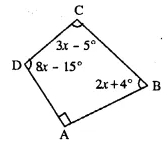Solution
∵ ∠A = 90˚ (Given)
∠B = (2x + 4˚)
∠C = (3x - 5˚)
∠D = (8x - 15˚)
∠A + ∠B + ∠C + ∠D = 360˚
90˚ + (2x + 4) + (3x – 5) + (8x – 15) = 360
⇒ 90 + 2x + 4 + 3x – 5 + 8x – 15 = 360
⇒ 74˚ + 13x  = 360˚
⇒ 13x = 360˚ - 74˚
⇒ 13x = 286˚
⇒ x = 22˚
∵  ∠B = 2x + 4 = 2×22˚ + 4 = 48˚
∠C = 3x – 5 = 3 × 22˚ - 5 = 61˚
Hence  (i)22˚  (ii) ∠B = 48˚, ∠C = 61˚

5. In quadrilateral ABCD, side AB is parallel to side DC. If ∠A : ∠D = 1 : 2 and ∠C : ∠B = 4 : 5
(i) Calculate each angle of the quadrilateral.
(ii) Assign a special name to quadrilateral ABCD
Solution∵ ∠A : ∠D = 1 : 2
Let ∠A = x and ∠B = 2x
∵ ∠C : ∠B  = 4 : 5
Let ∠C = 4y and ∠B = 5y
∵ AB ∥ DC
∴  ∠A + ∠D = 180˚
x + 2x = 180˚
⇒ 3x = 180˚
⇒ x = 60˚
∴ A = 60˚
∠D = 2x = 2 × 60˚ = 120˚
Again  ∠B + ∠C = 180˚
5y + 4y = 180˚
⇒ 9y = 180˚
⇒ y = 20˚
∴ ∠B = 5y = 5 × 20˚ = 100˚
∠C = 4y = 4 × 20˚ = 80˚
Hence ∠A = 60˚ : ∠B = 100˚  : ∠C = 80˚   ∠D = 120˚
(iii) Quadrilateral ABCD is a trapezium because one pair of opposite side is parallel.

6. From the following figure find ;
(i) x
(ii) ∠ABC
(iii) ∠ACD
Solution
x + 4x + 3x + 4x + 48° = 360°
⇒ 12x = 360° – 48°
⇒ 12x = 312
⇒ x = 312/12 = 26˚

(ii) ∠ABC  = 4x
4 × 26 = 104˚

(iii) ∠ACD = 180˚ - 4x - 48˚
= 180˚ - 4×26 - 48 ˚
= 180˚ - 104˚ - 48˚
= 180˚ - 152˚
= 28˚

7. Given : In quadrilateral ABCD ; ∠C = 64°, ∠D = ∠C – 8° ; ∠A = 5(a + 2)° and ∠B = 2(2a + 7)°.
Calculate ∠A.
Solution 7:
∠C = 64° (Given)
∠D = ∠C – 8° = 64° - 8° = 56°
∠A = 5(a + 2)°
∠B = 2(2a + 7)°
Now,  ∠A + ∠B + ∠C + ∠D = 360°
5(a + 2)° + 2(2a + 7)° + 64° + 56° = 360°
⇒ 5a + 10 + 4a + 14° + 64° + 56° = 360°
⇒ 9a + 144° = 360°
⇒ 9a = 360° – 144°
⇒ 9a = 216°
⇒ a = 24°
∠A = 5 (a + 2) = 5(24 + 2) = 130°

8. In the given figure : ∠b = 2a + 15 and ∠c = 3a + 5; find the values of b and c.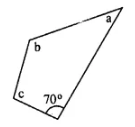Solution
Stun of angles of quadrilateral = 360°
70° + a + 2a + 15 + 3a + 5 = 360°
⇒ 6a + 90° = 360°
⇒ 6a = 270°
⇒ a = 45°
b = 2a + 15 = 2×45 + 15 = 105°
c = 3a + 5 = 3×45 + 5 = 140°
Hence ∠b and ∠c are 105° and 140°

9. Three angles of a quadrilateral are equal. If the fourth angle is 69°; find the measure of equal angles.
Solution
Let each equal angle be x°
x + x + x + 69° = 360°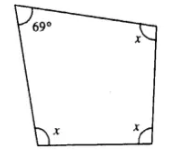3x = 360° - 69
⇒ 3x = 291
⇒ x = 97°
Each, equal angle = 97°

10. In quadrilateral PQRS, ∠P : ∠Q : ∠R : ∠S = 3 : 4 : 6 : 7.
Calculate each angle of the quadrilateral and then prove that PQ and SR are parallel to each other
(i) Is PS also parallel to QR?
(ii) Assign a special name to quadrilateral PQRS.
Solution
∵ ∠P : ∠Q + ∠R : ∠S = 3 : 4 : 5 : 6
Let  ∠P = 3x
∠Q = 4x
∠R = 6x
∠S = 7x
∴ ∠P + ∠Q + ∠R + ∠S = 360˚
⇒ 3x + 4x + 6x + 7x = 360˚
⇒ 20x = 360˚
⇒ x = 18˚
∴ ∠P = 3x = 3 × 18 = 54˚
∠Q = 4x = 4 × 18 = 72˚
∠R = 6x = 6 × 18 = 108˚
∠S = 7x = 7 × 18 = 126˚
∠Q + ∠R = 72˚ + 108˚ = 180˚
Or  ∠P + ∠S = 54˚ + 126 ˚ = 180˚
Hence PQ ∥ SR
As ∠P + ∠Q = 72˚ + 54˚ = 126˚
Which is ≠ 180˚
∴ PS and QR are not parallel.

(ii) PQRS is a  trapezium as its one pair of opposite side is parallel.

11. Use the information given in the following figure to find the value of x.Solution
Take A, B, C, D as the vertices of Quadrilateral and BA is produced to E (say).
∠DAB = 180° – 70° = 110°
[EAB is a straight line and AD stands on it ∠EAD + ∠DAB = 180°]
110° + 80° + 56° + 3x – 6° = 360°
[sum of interior angles of a quadrilateral = 360°]
3x = 360° – 110° – 80° – 56° + 6°
⇒ 3x = 360° – 240° = 120°
⇒ x = 40°

12. The following figure shows a quadrilateral in which sides AB and DC are parallel. If ∠A : ∠D = 4 : 5, ∠B = (3x – 15)° and ∠C = (4x + 20)°, find each angle of the quadrilateral ABCD.Solution
Let ∠A = 4x
∠D = 5x
Since ∠A + ∠D = 180° [AB || DC]
4x + 5x = 180°
⇒ 9x = 180°
⇒ x = 20°
∠A = 4 (20) = 80°,
∠D = 5 (20) = 100°
Again ∠B + ∠C = 180° [ AB || DC]
3x – 15° + 4x + 20° = 180°
⇒ 7x = 180° – 5°
⇒ 7x = 175°
⇒ x = 25°
∠B = 75° – 15° = 60°
and ∠C = 4(25) + 20 = 100° + 20° = 120°

13. Use the following figure to find the value of xSolution
The sum of exterior angles of a quadrilateral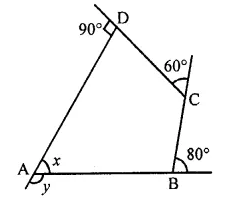y + 80° + 60° + 90° = 360°
⇒ y + 230° = 360°
⇒ y = 360° – 230° = 130°
At vertex A,
∠y + ∠x = 180° (Linear pair)
x = 180° – 130°
⇒ x = 50°

14. ABCDE is a regular pentagon. The bisector of angle A of the pentagon meets the side CD in point M. Show that ∠AMC = 90°.
Solution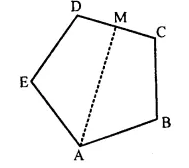Given: ABCDE is a regular pentagon.
The bisector ∠A of the pentagon meets the side CD at point M.
To prove: ∠AMC = 90°
Proof:
We know that, the measure of each interior angle of a regular pentagon is 108°.
∠BAM = 1/2 x 108° = 54°
Since, we know that the sum of a quadrilateral is 360°
∠BAM + ∠ABC + ∠BCM + ∠AMC = 360°
⇒ 54° + 108° + 108° + ∠AMC = 360°
⇒ ∠AMC = 360° – 270°
⇒ ∠AMC = 90°

15. In a quadrilateral ABCD, AO and BO are bisectors of angle A and angle B respectively. Show that:
∠AOB = 1/2 (∠C + ∠D)
Solution
Given: AO and BO are the bisectors of ∠A and ∠B respectively.
∠1 = ∠4 and ∠3 = ∠5 ...(i)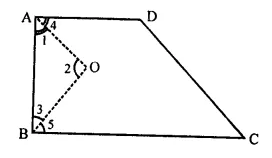To prove: ∠AOB = 1/2 (∠C + ∠D)
Proof: